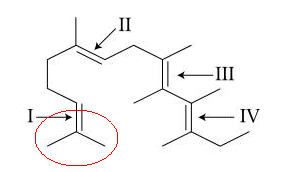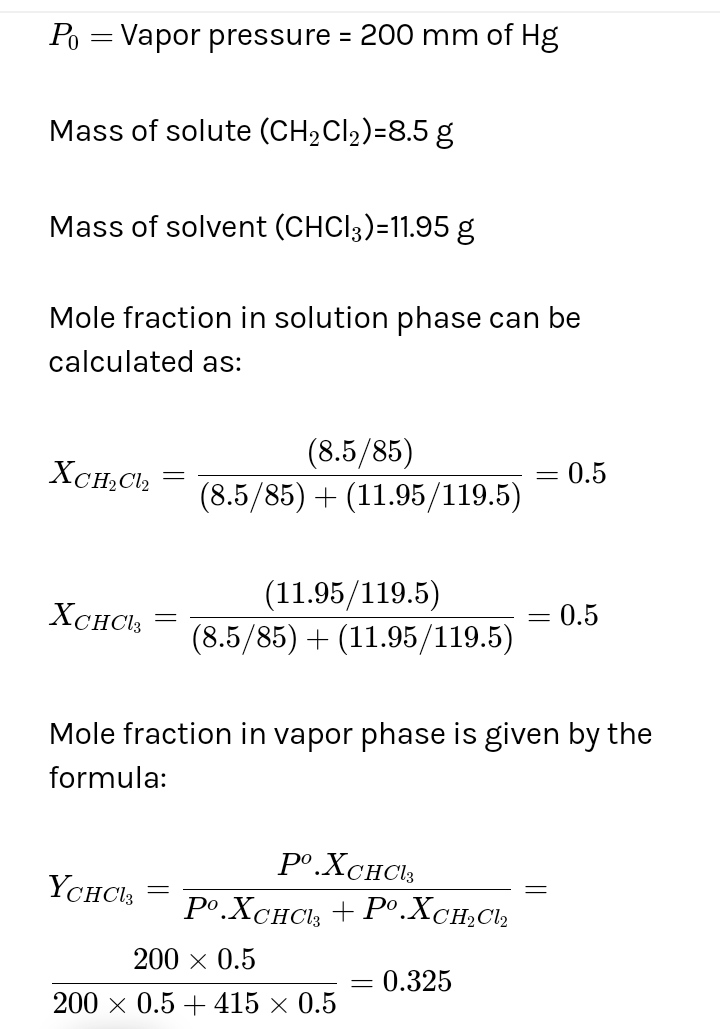Filter By

## All Questions

#### At 300 K and 1 atm, 15 mL of a gaseous hydrocarbon requires 375 mL air containing 20% O2 by volume for complete combustion.  After combustion, the gases occupy 330 mL.  Assuming that the water formed is in liquid form and the volumes were measured at the same temperature and pressure, the formula of the hydrocarbon is : Option: 1  C4H8   Option: 2  C4H10 Option: 3  C3H6 Option: 4  C3H8

Volume of N in air = 375 × 0.8 = 300 ml

Volume of O2 in air = 375 × 0.2 = 75 ml

15ml

0                         0                            15x                 -

After combustion total volume

330 = 300 + 15x

x = 2

Volume of O2 used

y = 12

So hydrocarbon is = C2H12

None of the options matches it therefore it is a BONUS.

----------------------------------------------------------------------

Alternatively  Solution

15ml

0                         0                            15x                 -

Volume of O2 used

If further information (i.e., 330 ml) is neglected, option (C3H8 ) only satisfy the above equation.

#### An ideal gas undergoes a quasi static, reversible process in which its molar heat capacity C remains constant.  If during  this process the relation of pressure P and volume V is given by PVn=constant,  then n is given by (Here CP and CV are molar specific heat at constant pressure and constant volume, respectively) Option: 1   Option: 2   Option: 3  Option: 4

For a polytropic preocess## Crack CUET with india's "Best Teachers"

• HD Video Lectures
• Unlimited Mock Tests
• Faculty Support#### A point particle of mass m, moves along the uniformly rough track PQR as shown in the figure.  The coefficient of friction, between the particle and the rough track equals µ.  The particle is released, from rest, from the point P and it comes to rest at a point R.  The energies, lost by the ball, over the parts, PQ and QR, of the track, are equal to each other, and no energy is lost when particle changes direction from PQ to QR. The values of the coefficient of friction µ and the distance x(=QR), are, respectively close to :Option: 1  0.2 and 6.5 m   Option: 3  0.2 and 3.5 m   Option: 4   0.29 and 6.5 m

Work done by friction at QR = μmgx

In triangle, sin 30° = 1/2 = 2/PQ

PQ = 4 m

Work done by friction at PQ = μmg × cos 30° × 4 = μmg × √3/2 × 4 = 2√3μmg

Since work done by friction on parts PQ and QR are equal,

μmgx = 2√3μmg

x = 2√3 ≅ 3.5 m

Applying work energy theorem from P to R

decrease in P.E.=P.E.= loss of energy due to friction in PQPQ and QR

where h=2(given)

#### In the following structure, the double bonds are marked as I, II, III and IVGeometrical isomerism is not possible at site (s) : Option: 1  III Option: 2  I Option: 3  I and II Option: 4  III and IV

Geometrical isomerism is not possible at Site I as two identical methyl groups are attached to the same carbon bearing the double bond.Hence, the answer is Option (2)

## Crack NEET with "AI Coach"

• HD Video Lectures
• Unlimited Mock Tests
• Faculty Support#### A particle of mass m is moving along the side of a square of side ‘a’, with a uniform speedin the x-y plane as shown in the figure :Which of the following statements is false for the angular momentumabout the origin? Option: 1 when the particle is moving from A to B. Option: 2 when the particle is moving from C to D. Option: 3 when the particle is moving from B to C. Option: 4when the particle is moving from D to A.

so option b is correct option

#### Which one of the following is an oxide ? Option: 1  KO2 Option: 2  BaO2 Option: 3  SiO2 Option: 4 CsO2SiO2  - oxide

KO???2? , CsO????2  -  superoxides

BaO????2 -  peroxide

## Crack JEE Main with "AI Coach"

• HD Video Lectures
• Unlimited Mock Tests
• Faculty Support#### The following reaction occurs in the Blast Furnace where iron ore is reduced to iron metal : Fe2O3(s)+3 CO(g) 2 Fe(l)+3 CO2(g) Using the Le Chatelier’s principle, predict which one of the following will not disturb the equilibrium ? Option: 1  Removal of CO Option: 2  Removal of CO2 Option: 3 Addition of CO2 Option: 4 Addition of Fe2O3According to Le Chatelier's principle change in concentration by changing the amount of reactant or product affect the equilibrium. However, the addition of solid reactant won't affect the concentration.

Therefore, addition of solid Fe2O???3 will not disturb the equilibrium.

#### The electronic configuration with the highest ionization enthalpy is : Option: 1  [Ne] 3s2 3p1 Option: 2  [Ne] 3s2 3p2 Option: 3  [Ne] 3s2 3p3 Option: 4 [Ar] 3d10 4s2 4p3

The electronic configuration with the highest ionization enthalpy is [Ne]3s23p3. On moving down the group, the  ionization enthalpy decreases. In a period, on moving from left tor fight, the  ionization enthalpy increases.

## Crack CUET with india's "Best Teachers"

• HD Video Lectures
• Unlimited Mock Tests
• Faculty Support#### A solution is prepared by mixing 8.5 g of CH2Cl2 and 11.95 g of CHCl3.  If vapour pressure of CH2Cl2 and CHCl3 at 298 K are 415 and 200 mmHg respectively, the mole fraction of CHCl3 in vapour form is : (Molar mass of Cl=35.5 g mol−1)   Option: 1  0.162 Option: 2 0.675 Option: 3 0.325 Option: 4  0.486#### What quantity (in mL) of a 45% acid solution of a monoprotic strong acid must be mixed with a 20% solution of the same acid to produce 800 mL of a 29.875% acid solution? Option: 1 320 Option: 2 325 Option: 3 316 Option: 4 330

Reactions in Solutions -

Concentration :
It is the amount of solute present in one litre of solution. It is denoted by C or S.Mole Fraction:
It is the ratio of moles of one component to the total number of moles present In the solution. It is expressed by X for example, for a binary solution with two components A and B.Here nA and nB represent moles of solvent and solute respectively. Mole fraction does not depend upon temperature as both solute and solvent are expressed by weight.

Molarity:
It is the number of moles or gram moles of solute dissolved per litre of the solution. Molarity is denoted by 'M'.• When molarity of a solution is one, it is called a molar solution and when it is 0.1, solution is called decimolar solution.
•  Molarity depends upon temperature and its unit is mol/litre.
M1V1 = M2V2
• On dilution water added = V2 - V1
• When the solution of two different substances react together thenHere M, V, n are molarity, volume and number of molecules taking part in a reaction respectively.
• When a mixture of different solutions having different concentrations are taken the molarity of the mixture is calculated as follows:• When density and % by weight of a substance in a solution are given, molarity is find as follows:Here d = density

Molality
It is the number of moles or gram moles of solute dissolved per kilogram of the solvent. It is denoted by 'm'.• If molality is one solution, it is called molal solution.
• One molal solution is less than one molar solution.
• Molality is preferred over molarity during experiments as molality is temperature independent while molarity is temperature-dependent.

Normality
It is the number of gram equivalents of solute present in one litre of the solution and it is denoted by 'N'.• When normality of a solution is one, the solution is called normal solution and when it is 0.1, the solution is called deci-normal solution.

Normality Equation:• Volume Of water added = V2 - V1
Here V2 = volume after dilution
V1 = volume before dilution
• When density and % by weight of a substance in a solution are given, normality is find as follows:Here d = density of solution
• When a mixture of different solutions having different concentrations are taken the normality of the mixture is calculated as follows:• In case of acid-base neutralization the normality of the resulting solution• To find weight of substanceRelation between Normality and Molarity :
N x Eq wt. = molarity x molar mass
N = molarity x valency
N = molarity x number of H+ or OH- ion

-

As we have learnt,Therefore, Option (3) is correct